pH, pOH, and K

pH and pOH

Adding an acid to water increases the H3O+ ion concentration and decreases the OH- ion concentration. Adding a base does the opposite. Regardless of what is added to water, however, the product of the concentrations of these ions at equilibrium is always 1.0 x 10-14 at 25oC.

[H3O+][OH-] = 1.0 x 10-14

The table below lists pairs of H3O+ and OH- ion concentrations that can coexist at equilibrium in water at 25oC.

Pairs of Equilibrium Concentrations of H3O+ and OH- Ions That Can Coexist in Water

 Concentration (mol/L) [H3O+] [OH-] 1 1 x 10-14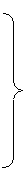1 x 10-1 1 x 10-13 1 x 10-2 1 x 10-12 1 x 10-3 1 x 10-11 Acidic Solution 1 x 10-4 1 x 10-10 1 x 10-5 1 x 10-9 1 x 10-6 1 x 10-8 1 x 10-7 1 x 10-7 Neutral Solution 1 x 10-8 1 x 10-61 x 10-9 1 x 10-5 1 x 10-10 1 x 10-4 1 x 10-11 1 x 10-3 Basic Solution 1 x 10-12 1 x 10-2 1 x 10-13 1 x 10-1 1 x 10-14 1

Data from this table are plotted in the figure below over a narrow range of concentrations between 1 x 10-7 M and 1 x 10-6 M. The point at which the concentrations of the H3O+ and OH- ions are equal is called the neutral point. Solutions in which the concentration of the H3O+ ion is larger than 1 x 10-7 M are described as acidic. Those in which the concentration of the H3O+ ion is smaller than 1 x 10-7 M are basic.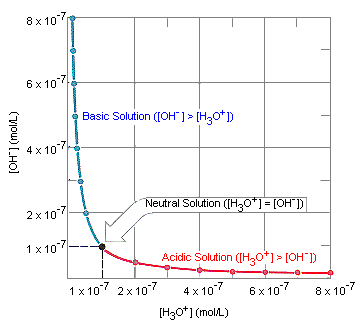It is impossible to construct a graph that includes all the data from the table given above. In 1909, the Danish biochemist S. P. L. Sorenson proposed using logarithmic mathematics to condense the range of H3O+ and OH- concentrations to a more convenient scale. By definition, the logarithm of a number is the power to which a base must be raised to obtain that number. The logarithm to the base 10 of 10-7 for example, is -7.

log (10-7) = -7

Since the concentrations of the H3O+ and OH- ions in aqueous solutions are usually smaller than 1 M, the logarithms of these concentrations are negative numbers. Because he considered positive numbers more convenient, Sorenson suggested that the sign of the logarithm should be changed after it had been calculated. He therefore introduced the symbol "p" to indicate the negative of the logarithm of a number. Thus, pH is the negative of the logarithm of the H3O+ ion concentration.

pH = - log [H3O+]

Similarly, pOH is the negative of the logarithm of the OH- ion concentration.

pOH = - log [OH-]

pH + pOH = 14

The equation above can be used to convert from pH to pOH, or vice versa, for any aqueous solution at 25C, regardless of how much acid or base has been added to the solution. By converting the H3O+ and OH- ion concentrations in the table above into pH and pOH data, we can fit the entire range of concentrations onto a single graph, as shown in the figure below.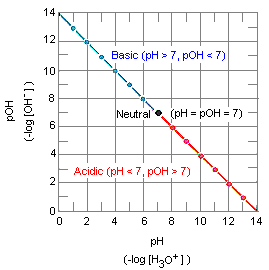Acid-Dissociation Equilibrium Constants

There is a big difference between strong acids such as hydrochloric acid and weak acids such as the acetic acid in vinegar. Both compounds satisfy the Brnsted definition of an acid. (They are both H+ ion, or proton, donors.) But they differ in the extent to which they donate H+ ions to water.

By definition, a strong acid is any substance that is good at donating an H+ ion to water.

Example: 99.996% of the HCl molecules in a 6 M solution dissociate when the following reaction comes to equilibrium. This equilibrium lies so far to the right that we write the equation for the reaction with a single arrow, suggesting that hydrochloric acid dissociates more or less completely in aqueous solution.

 HCl(aq) + H2O(l)H3O+(aq) + Cl-(aq)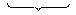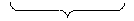0.004% 99.996% at equilibrium at equilibrium

Weak acids are relatively poor H+ ion donors.

Example: Acetic acid is a Brnsted acid because it can donate an H+ ion to water. But it isn't a very good H+ ion donor. Only about 1.3% of the acetic acid molecules in an 0.10 M solution lose a proton to water.

 CH3CO2H(aq) + H2O(l )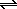H3O+(aq) + CH3CO2-(aq)98.7% 1.3% at equilibrium at equilibrium

A quantitative feeling for the difference between strong acids and weak acids can be obtained from the equilibrium constants for the reactions between acids and water. Because it is time-consuming to write the formula CH3CO2H for acetic acid, chemists commonly abbreviate this formula as HOAc and describe the dissociation of the acid as follows.

HOAc(aq) + H2O(l)H3O+(aq) + OAc-(aq)

Using this convention, the equilibrium constant expression for the reaction between acetic acid and water would be written as follows.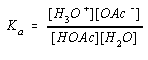Like the equilibrium constant expression for the dissociation of water, this is a legitimate equation. But most acids are weak, so the equilibrium concentration of H2O is effectively the same after dissociation as before the acid was added. Because the [H2O] term has no effect on the equilibrium it is built into the equilibrium constant for the reaction as follows.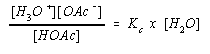The result is an equilibrium constant for this equation known as the acid-dissociation equilibrium constant, Ka. For this reaction: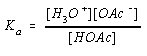In general, for any acid HA: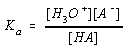Values of Ka can be used to estimate the relative strengths of acids. The larger the value of Ka, the stronger the acid. By definition, a compound is classified as a strong acid when Ka is larger than 1. Weak acids have values of Ka that are smaller than 1. A list of the acid-dissociation equilibrium constants for some common acids is given in the table below.

Values of Ka for Common Acids

 Strong Acids Ka hydrochloric acid (HCl) 1 x 106 sulfuric acid (H2SO4) 1 x 103 hydronium ion (H3O+) 55 nitric acid (HNO3) 28 Weak Acids Ka phosphoric acid (H3PO4) 7.1 x 10-3 citric acid (C6H7O8) 7.5 x 10-4 acetic acid (CH3CO2H) 1.8 x 10-5 boric acid (H3BO3) 7.3 x 10-10 water (H2O) 1.8 x 10-16

The table above provides us with the basis for understanding the difference between strong acids and weak acids. Think about the reaction between a very strong acid and water.

 HCl(aq) + H2O(l)H3O+(aq) + Cl-(aq) Ka = 106 Ka = 55

HCl is a much stronger acid than the H3O+ ion. This means that H2O is a stronger base than the Cl- ion. It isn't surprising to find that the stronger of a pair of acids reacts with the stronger of a pair of bases to give a weaker acid and a weaker base.

Let's consider the reaction between acetic acid and water.

 HOAc(aq) + H2O(l)H3O+(aq) + OAc-(aq) Ka = 1.8 x 10-5 Ka = 55

In this case, the reaction tries to convert the weaker of a pair of acids and the weaker of a pair of bases into a stronger acid and a stronger base. It isn't surprising to find that this reaction occurs to only a minor extent.

As the value of Ka decreases further the extent to which the acid will react with water must decrease as well. Inevitably, we should encounter acids that are so weak they can't compete with water as a source of the H3O+ ion.